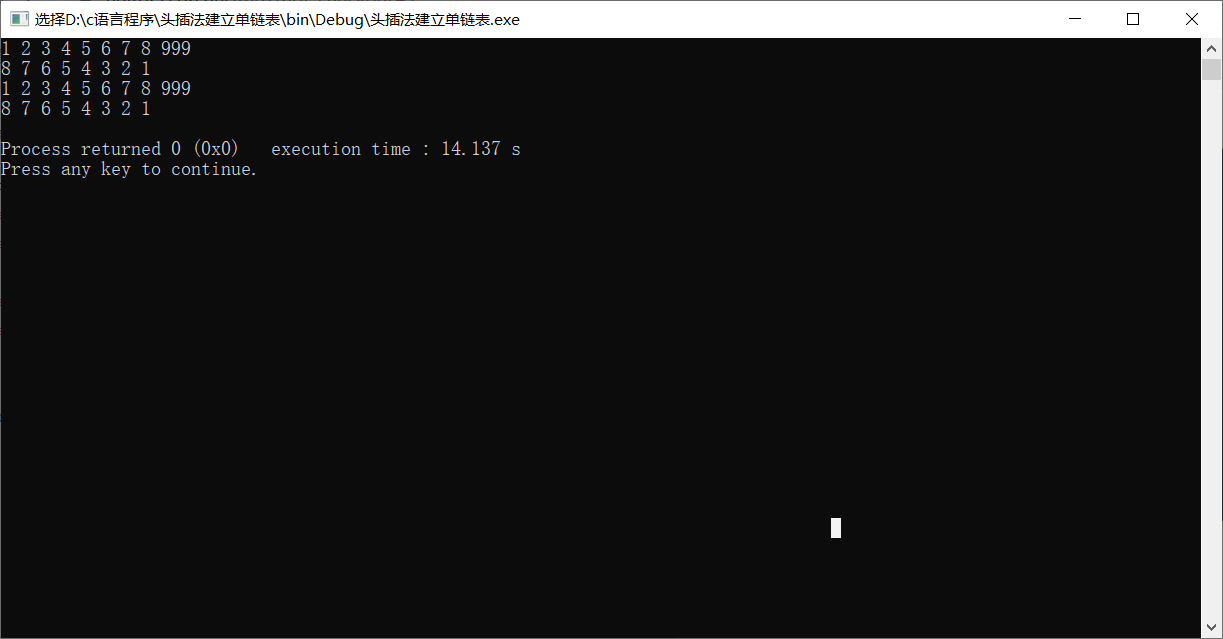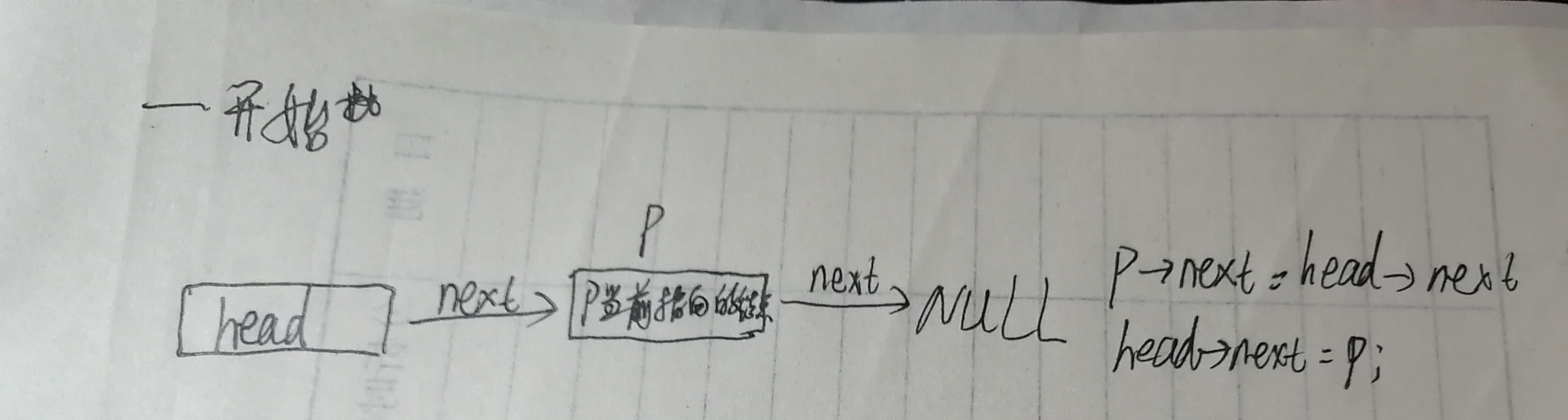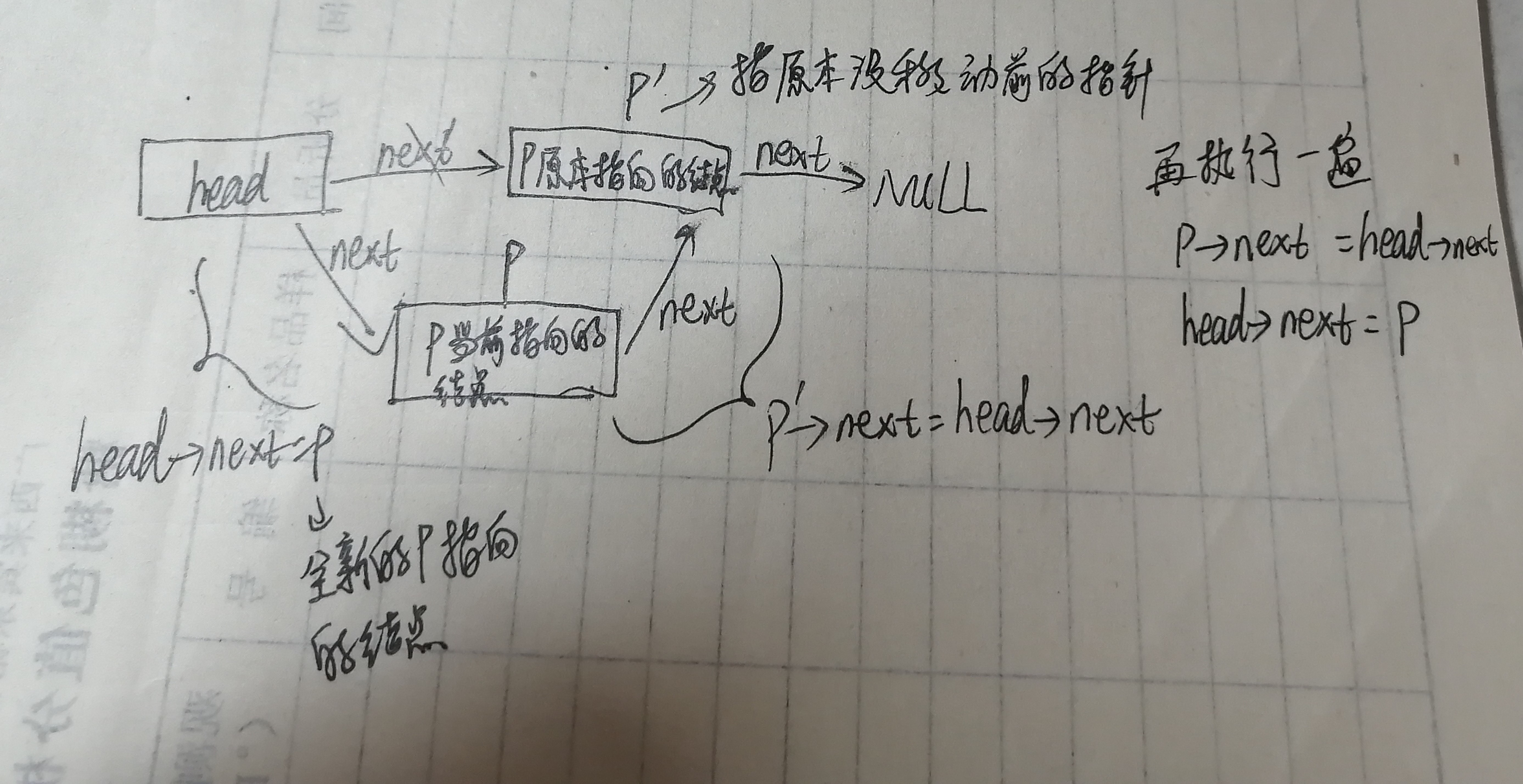• #include<bits/stdc++.h>...//头插法建立带头结点的单链表 void ListHeadInsert(LNode * &L){ int value; LNode *p; L=(LNode *)malloc(sizeof(LNode)); L->next=NULL; cin>
#include<bits/stdc++.h>
using namespace std;

struct LNode{
int data;
LNode *next;
};

//头插法建立带头结点的单链表

int value;
LNode *p;
L=(LNode *)malloc(sizeof(LNode));
L->next=NULL;

cin>>value;

while(value!=999){

p=(LNode *)malloc(sizeof(LNode));
p->data=value;
p->next=L->next;
L->next=p;

cin>>value;
}

}

//遍历带头结点的单链表
void ListShow(LNode *L){

LNode *T=L->next;
while(T!=NULL){

cout<<T->data<<" ";
T=T->next;
}
cout<<endl;
}

//头插法建立没有头节点的单链表

int value,flag=1;
LNode *p;

cin>>value;

while(value!=999){

if(flag){
L=(LNode *)malloc(sizeof(LNode));
L->data=value;
L->next=NULL;
flag=0;
}else{
p=(LNode *)malloc(sizeof(LNode));
p->data=value;
p->next=L;
L=p;
}

cin>>value;
}

}

//遍历没有头节点的链表
void ListShow2(LNode *L){

LNode *T=L;
while(T!=NULL){

cout<<T->data<<" ";
T=T->next;
}
cout<<endl;
}

int main(){

LNode *L,*nL;
ListShow(L); //遍历带头节点的单链表

ListShow2(nL); //遍历没有头节点的单链表

return 0;
}展开全文链表 数据结构
• 首先要明确一点，利用头插法建立出来的单链表的输出都是逆序的（就是和你的输入顺序反着来的）然后就是要明确生成的新结点是一个个加在头结点的前面的，这就是头插法。至于怎么将结点一个个插入在头结点前面呢？下面...
      首先要明确一点，利用头插法建立出来的单链表的输出都是逆序的（就是和你的输入顺序反着来的）然后就是要明确生成的新结点是一个个加在某个结点的前面的（这个结点不一定是头结点，下面的代码是插在p的前头），这就是头插法。至于怎么将结点一个个插入在某个结点前面呢？下面的图可以比较详细的展示出来（至少对我来说挺详细的哈)

以下是图像展示：以下是图像展示：然后就是不断地重复上述过程，在头节点和新插入的节点之间建立联系，同时在新插入的节点和上一个插入的节点之间建立联系，最终得到一个完整的单链表。
以下是代码展示：
#include <bits/stdc++.h>

using namespace std;
//利用头插法建立的单链表输出都是逆序的
typedef struct node
{
int data;//数据域，存放数据
struct node *next;//指针域，存放指针，存放后继结点的信息
}no;//no是结构体的名字

int main()
{
int n;
cin>>n;
while(n)
{
p=new no;
p->data=n;
//再往后，指的就是头结点后面的结点
cin>>n;
}
while(p!=NULL)
{
printf("%d ",p->data);
p=p->next;
}
printf("\n");
return 0;
}


展开全文• 使用头插法建立单链表并输出## 标题 # include<stdio.h> typedef struct node{ int data; struct node *next; } Lnode; Lnode *Head_Insert(){ Lnode *ptemp = NULL; Lnode *HeadNode = NULL; Lnode *p...
使用头插法建立单链表并输出## 标题
# include<stdio.h>

typedef struct node{
int data;
struct node *next;
} Lnode;

Lnode *ptemp = NULL;
Lnode *p;//前面这里是声明这里有多少个变量
int x;
scanf("%d",&x);
while(x != 9999){
ptemp = (Lnode*)malloc(sizeof(Lnode));
ptemp->data = x;
ptemp->next = NULL;
} else{

}
scanf("%d",&x);
}
}

void printList(Lnode *L){//拿到这个头结点
L = L->next;//拿到头结点
while(L!=NULL){//判断头结点是否为空
printf("%d ",L->data);
L = L->next;
}
}
int main(){
printList(t);
return 0;
}


刚学习C语言，C语言小白，中间有一些地方需要注意
Main需要定义在自定义函数的下面，或者说应该可以将Main函数写在上面，但是需要在里面写出定义了那些方法。返回值如果是一个指针的话，那么在声明方法的时候该方法也应该加上指针符号。
展开全文c语言 指针 链表
• #include <iostream> typedef struct LNode { int data; struct LNode *next; } LNode; void createList(LNode *&C, int arr[], int n) { LNode *s; int i; C = (LNode *) malloc(sizeof... i ...
#include <iostream>
typedef struct LNode {     int data;     struct LNode *next; } LNode;
void createList(LNode *&C, int arr[], int n) {     LNode *s;     int i;     C = (LNode *) malloc(sizeof(LNode));     C->next = NULL;     for (i = 0; i < n; ++i) {         s = (LNode *) malloc(sizeof(LNode));         s->data = arr[i];         s->next = C->next;         C->next = s;     } };
int main() {     int arr[] = {1, 2, 5, 1, 8, 34, 5, 4};     int n = 8;     LNode *c;     createList(c, arr, n);     return 0; }
展开全文数据结构
• 头插法创建单链表，可输入式，数据结构的基本操作，C++代码实现数据结构
• //头插法，s的指针指向c的头结点的指针 void createlist ( Llist * & c , int a [ ] , int length ) { Llist * s ; c = ( Llist * ) malloc ( sizeof ( Llist ) ) ; c -> next = NULL ; //把c的指针...
• #include <stdio.h> #include <stdlib.h> typedef struct LNode { int data; struct LNode *next; }LNode,*LinkList; LinkList L;//全局变量L，可以省略函数参数... LinkList insert()//头插法 { ...
• /* L是带头结点的空链表头指针，通过键盘输入表中元素值，利用头插法单链表L*/ { Node *s; char c; int flag = 1; while (flag) // flag初值为1，当输入“\$”时，置flag为0，建表结束 { c = getchar(); ...数据结构
• 1、采用头插法建立单链表 2、尾插法建立单链表 3、按序号查找结点值 4、按值查找表结点 5、插入结点操作 6、删除操作 7、求表长 本篇博客的结点数据结构如下： typedef struct LNode { ElemType data; ...算法 数据结构 c++
•c语言
• typedef struct LNode{ //单链表结点的定义 int data; struct LNode *next; }LNode,*LinkList; int main(){ int i=1,x,N; printf("插入的数字个数："); scanf("%d",&N); printf("依次输入各数字："); ...数据结构 指针 算法
• #include #include #include struct ListNode { char data;//数据域 struct ListNode* next;//指针域 };//头插法建立单链表 struct ListNode*CreateList() { char ch; struct ListN
• 头插法建立单链表的算法 实现代码： #include #include using namespace std; #define maxSize 100 typedef struct LNode{ int data; struct LNode *next; }LNode; void createListR(LNode *&C,int a[],
• 【算法代码】 #include<iostream> using namespace std; typedef struct LNode { ...L,int n) { //头插法 L=new LNode; //生成头结点，用头指针L指向它 L->next=NULL; for(int i=0; i<n; i++)数据结构
• 今天和鹏学长研究了很长时间头插法单链表，总的来说思路还是明确的，主要是对代码的编写能力还是有待提高，先贴上第一个问题的起初代码：#include #include <stdlib.h>typedef int DataType; typedef struct node {...c语言
• 编译通过，逻辑问题我也不知道出在哪里，修改了好几次都不能正常运行链表
•代码
• #include <iostream> #include <stdlib.h> #include <cstdio> typedef int ElemType; using namespace std; //单链表的结构体 ...//头插法建立单链表 LinkList List_HeadInsert(LinkList &....